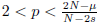#### 期刊菜单

Existence of Sign-Changing Solutions for a Fractional Choquard Equation
DOI: 10.12677/AAM.2022.117437, PDF, 下载: 56  浏览: 106

(−∆)su + V (x)u = (|x|−µ ∗ |u|p)|u|p−2u, x ∈ RN , (P)

Abstract:

With an important physical background, the fractional Choquard equation has at- tracted great attention from the field of nonlinear analysis in recent years. In this paper, we study the following fractional Choquard equation

(−∆)su + V (x)u = (|x|−µ ∗ |u|p)|u|p−2u, x ∈ RN , (P)

where s ∈ (0, 1), N ≥ 3, µ ∈ (0, N ),, “∗” stands for the convolution and (−∆)s is the fractional Laplacian operator. By combining the Ekeland variational principle with the implicit function theorem, we prove that the problem (P ) possesses one least energy sign-changing solution w. Moreover, we show that the energy of w is strictly larger than the ground state energy and less than twice the ground state energy.

  Lieb, E. (1977) Existence and Uniqueness of the Minimizing Solution of Choquard’s Nonlinear Equation. Studies in Applied Mathematics, 57, 93-105. https://doi.org/10.1002/sapm197757293  Pekar, S. (1954) Untersuchung Berlin. u¨ber die elektronentheorie der kristalle. Akademie Verlag,  Moroz, I., Penrose, R. and Tod, P. (1998) Spherically-Symmetric Solutions of the Schro¨dinger- Newton Equations. Classical and Quantum Gravity, 15, 2733-2742. https://doi.org/10.1088/0264-9381/15/9/019  Bahrami, M., Großardt, A., Donadi, S. and Bassi, A. (2014) The Schro¨dinger-Newton Equation and Its Foundations. New Journal of Physics, 16, 7-28.https://doi.org/10.1088/1367-2630/16/11/115007  Giulini, D. and Großardt, A. (2012) The Schro¨dinger-Newton Equation as a Non-Relativistic Limit of Self-Gravitating Klein-Gordon and Dirac Fields. Classical and Quantum Gravity, 29, Article ID: 215010. https://doi.org/10.1088/0264-9381/29/21/215010  Moroz, V. and Schaftingen, J.V. (2017) A Guide to the Choquard Equation. Journal of Fixed Point Theory and Applications, 19, 773-813. https://doi.org/10.1007/s11784-016-0373-1  Alves, C., N´obrega, A. and Yang, M. (2016) Multi-Bump Solutions for Choquard Equation with Deepening Potential Well. Calculus of Variations and Partial Differential Equations, 55, 1-28. https://doi.org/10.1007/s00526-016-0984-9  Moroz, V. and Schaftingen, J.V. (2013) Groundstates of Nonlinear Choquard Equations: Ex- istence, Qualitative Properties and Decay Asymptotics. Journal of Functional Analysis, 265, 153-184. https://doi.org/10.1016/j.jfa.2013.04.007  Moroz, V. and Schaftingen, J.V. (2015) Existence of Groundstates for a Class of Nonlinear Choquard Equations. Transactions of the American Mathematical Society, 367, 6557-6579. https://doi.org/10.1090/S0002-9947-2014-06289-2  Gao, F. and Yang, M. (2017) On Nonlocal Choquard Equations with Hardy-Littlewood- Sobolev Critical Exponents. Journal of Mathematical Analysis and Applications, 448, 1006- 1041. https://doi.org/10.1016/j.jmaa.2016.11.015  Clapp, M. and Salazar, D. (2013) Positive and Sign Changing Solutions to a Nonlinear Choquard Equation. Journal of Mathematical Analysis and Applications, 407, 1-15. https://doi.org/10.1016/j.jmaa.2013.04.081  Ye, H. (2015) The Existence of Least Energy Nodal Solutions for Some Class of Kirchhoff Equations and Choquard Equations in RN . Journal of Mathematical Analysis and Applications, 431, 935-954. https://doi.org/10.1016/j.jmaa.2015.06.012  Ghimenti, M. and Schaftingen, J.V. (2016) Nodal Solutions for the Choquard Equation. Jour- nal of Functional Analysis, 271, 107-135. https://doi.org/10.1016/j.jfa.2016.04.019  Ghimenti, M., Moroz, V. and Schaftingen, J.V. (2017) Least Action Nodal Solutions for the Quadratic Choquard Equation. Proceedings of the American Mathematical Society, 145, 737- 747. https://doi.org/10.1090/proc/13247  d’Avenia, P., Siciliano, G. and Squassina, M. (2015) Existence Results for a Doubly Nonlocal Equation. Sao Paulo Journal of Mathematical Sciences, 9, 311-324. https://doi.org/10.1007/s40863-015-0023-3  d’Avenia, P., Siciliano, G. and Squassina, M. (2015) On Fractional Choquard Equations. Math- ematical Models and Methods in Applied Sciences, 25, 1447-1476. https://doi.org/10.1142/S0218202515500384  Chen, Y. and Liu, C. (2016) Ground State Solutions for Non-Autonomous Fractional Choquard Equations. Nonlinearity, 29, 1827-1842. https://doi.org/10.1088/0951-7715/29/6/1827  Shen, Z., Gao, F. and Yang, M. (2016) Groundstates for Nonlinear Fractional Choquard E- quations with General Nonlinearities. Mathematical Models and Methods in Applied Sciences, 39, 4082-4098. https://doi.org/10.1002/mma.3849  Wei, S. (2015) Sign-Changing Solutions for a Class of Kirchhoff-Type Problem in Bounded Domains. Journal of Differential Equations, 259, 1256-1274. https://doi.org/10.1016/j.jde.2015.02.040  Lieb, E. and Loss, M. (1997) Analysis, Second Edition. In: Graduate Studies in Mathematics, Vol. 14, American Mathematical Society, Providence, RI.  Nezza, E., Palatucci, G. and Valdinoci, E. (2012) Hitchhiker’s Guide to the Fractional Sobolev Spaces. Bulletin des Sciences Math´ematiques, 136, 521-573. https://doi.org/10.1016/j.bulsci.2011.12.004  Caffarelli, L. and Silvestre, L. (2006) An Extension Problem Related to the Fractional Lapla- cian. Communications in Partial Differential Equations, 32, 1245-1260. https://doi.org/10.1080/03605300600987306  Br¨andle, C., Colorado, E., Pablo, A. and S´anchez, U. (2010) A Concave-Convex Elliptic Prob- lem Involving the Fractional Laplacian. Proceedings of the Royal Society of Edinburgh Section A: Mathematics, 143, 39-71. https://doi.org/10.1017/S0308210511000175  Castro, A., Cossio, J. and Neuberger, J. (1997) A Sign-Changing Solution for a Superlinear Dirichlet Problem. The Rocky Mountain Journal of Mathematics, 27, 1041-1053. https://doi.org/10.1216/rmjm/1181071858  Bartsch, T., Weth, T. and Willem, M. (2005) Partial Symmetry of Least Energy Nodal Solu- tions to Some Variational Problems. Journal d’Analyse Math´ematique, 96, 1-18. https://doi.org/10.1007/BF02787822  Weth, T. (2006) Energy Bounds for Entire Nodal Solutions of Autonomous Superlinear Equa- tions. Calculus of Variations and Partial Differential Equations, 27, 421-437. https://doi.org/10.1007/s00526-006-0015-3  Bartsch, T. and Weth, T. (2005) Three Nodal Solutions of Singularly Perturbed Elliptic Equa- tions on Domains without Topology. Annales de l’Institut Henri Poincar´e C, Non Lin´eaire, 22, 259-281. https://doi.org/10.1016/j.anihpc.2004.07.005  Willem, M. (1996) Minimax Theorems. Springer Science + Business Media, Berlin. https://doi.org/10.1007/978-1-4612-4146-1